### IMO Shortlist 2000 problem G6

Kvaliteta:
Avg: 0,0
Težina:
Avg: 8,0
Let$ABCD$ be a convex quadrilateral. The perpendicular bisectors of its sides$AB$ and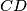$CD$ meet at$Y$. Denote by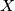$X$ a point inside the quadrilateral$ABCD$ such that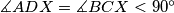$\measuredangle ADX = \measuredangle BCX < 90^{\circ}$ and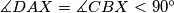$\measuredangle DAX = \measuredangle CBX < 90^{\circ}$. Show that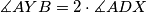$\measuredangle AYB = 2\cdot\measuredangle ADX$.
Izvor: Međunarodna matematička olimpijada, shortlist 2000